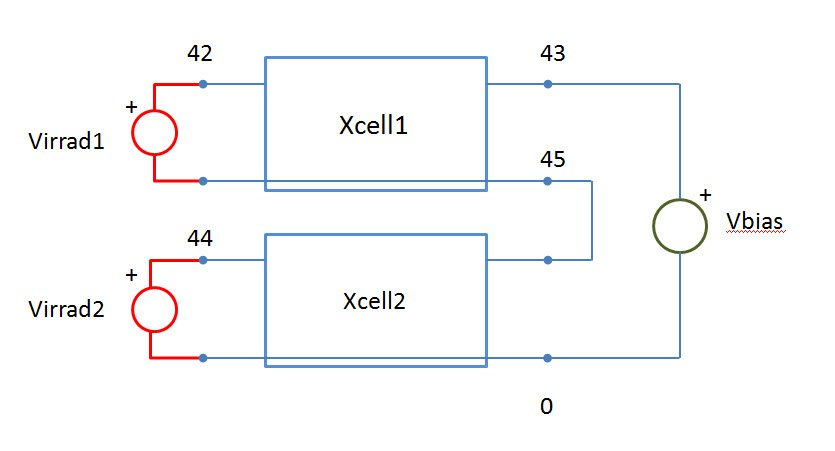# Problems with Spice circuit

Home Forums Photovoltaic Circuits Problems with Spice circuit

• This topic is empty.
Viewing 5 posts - 1 through 5 (of 5 total)
• Author
Posts
• #16322raheemq
Guest

Hi guys,
I’m basically new to the PSpice software and I got to do project to my engineering class. The thing is the I’ve got .cir file, which is:
* cell_3.cir
.include elementy.lib
xcell1 45 43 42 cell_2 params:area=126.6 j0=1e-11 j02=1E-9
+jsc=0.0343 RSH=1000 RS=0.0001
xcell2 0 45 44 cell_2 params:area=126.6 j0=1e-11 j02=1E-9
+jsc=0.0343 RSH=1000 RS=0.0001
vbias 43 0 dc 0
.probe
.plot dc i(vbias)
.dc vbias 0 2 0.01
.end

Subcircuit is:
.subckt cell_1 300 301 302 params: area=1, j0=1, jsc=1
d1 301 300 diode
.model diode d(is={j0*area})
.ends cell_1
.subckt cell_2 300 303 302 params:area=1, j0=1, jsc=1, j02=1, RSH=1, RS=1
d1 301 300 diode
.model diode d(is={j0*area})
d2 301 300 diode2
.model diode2 d(is={j02*area}, n=2)
Rsh 301 300 {RSH}
Rs 301 303 {RS}
.ends cell_2

And now I’ve got to add one more module in a serial way. And how am I supposed to do it?
It should be of course additional:
xcell3 0 45 44 cell_2 params:area=126.6 j0=1e-11 j02=1E-9
+jsc=0.0343 RSH=1000 RS=0.0001

#16471

Hi raheemq,

Virrad, in the SPICE model, is a voltage, but actually it represents numerically the value of the Irradiance.
The irradiance is measured in W/m^2 and considers the contributions of all wavelengths to the power that affects a surface.
As a first approximation, an ideal solar cell can be modelled in SPICE by a voltage controlled current source, that receives an Irradiance (represented by Virrad) and provides a short circuit current proportional to the irradiance, and a diode that represents the open circuit voltage. (subcircuit cell_1).
cell_3.cir refers to cell_2 subcircuit that is a generalized SPICE model of a solar cell that includes series and shunt resistance and two diodes that model other important electrical characteristics of the solar cell.
If you want add a third series solar cell you can do for example:

xcell1 45 43 42

xcell2 47 45 44

xcell3 0  47 46

#16472raheemq
Guest

Hi Cribald.
First of all thank you for your help!
I did as you wrote, but still I’ve got a problem. Now my circuit looks:
* cell_3.cir
.include elementy.lib
xcell1 45 43 42 cell_2 params:area=126.6 j0=1e-11 j02=1E-9
+jsc=0.0343 RSH=1000 RS=0.0001

xcell2 47 45 44 cell_2 params:area=126.6 j0=1e-11 j02=1E-9
+jsc=0.0343 RSH=1000 RS=0.0001

xcell3 0 47 46 cell_2 params:area=126.6 j0=1e-11 j02=1E-9
+jsc=0.0343 RSH=1000 RS=0.0001

vbias 43 0 dc 0
.probe
.plot dc i(vbias)
.dc vbias 0 2 0.01

.end

and I’d like to take trace of I(vbias) for the whole system. First of all current is negative (why?) and changing virrad of any solar cell do not change the current. And as far as I know it should affect the current, am I right?

#16473